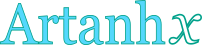## Inverse hyperbolic tangent calculator

This tool evaluates the inverse hyperbolic tangent of a number: artanh(x). Enter the argument x below.

 x = Result: artanh(x) =## Definitions

### General

The inverse hyperbolic tangent function, in modern notation written as artanh(x) or arctanh(x) or tanh-1x, gives the value t (hyperbolic angle), so that:

The inverse hyperbolic tangent function accepts arguments in real open interval (-1,1), because . Since the hyperbolic tangent is defined through the natural exponential function , its inverse can be defined through the natural logarithm function, using the following formula, for real x, with |x|<1:

### Properties

The derivative of the inverse hyperbolic tangent function is:

The integral of the inverse hyperbolic tangent function is given by: